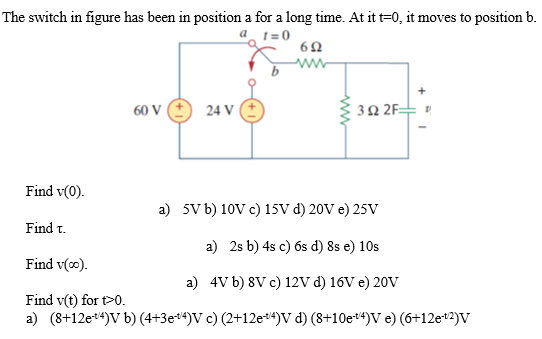# (Solved): i dont a book alike answer...its fully of equations that the author assumes a student knows and othe ...The switch in figure has been in position a for a long time. At it $$t=0$$, it moves to position $$b$$. Find $$v(0)$$. Find $$\tau$$. a) $$5 \mathrm{~V} \mathrm{~b} 10 \mathrm{~V}$$ c) $$15 \mathrm{~V}$$ d) $$20 \mathrm{~V} \mathrm{e}) 25 \mathrm{~V}$$ Find $$v(\infty)$$. a) $$2 \mathrm{~s}$$ b) $$4 \mathrm{sc}$$ c) $$6 \mathrm{~s}$$ d) $$8 \mathrm{se}$$ e) $$10 \mathrm{~s}$$ Find $$v(t)$$ for $$t>0$$. a) $$4 \mathrm{~V}$$ b) $$8 \mathrm{~V} \mathrm{c}$$ c) $$12 \mathrm{~V} \mathrm{~d}$$ ) $$16 \mathrm{~V} \mathrm{e}$$ ) $$20 \mathrm{~V}$$ a) $$\left.\left.\left.\left.\left(8+12 \mathrm{e}^{-t / 4}\right) \mathrm{V} \mathrm{b}\right)\left(4+3 \mathrm{e}^{-t 4}\right) \mathrm{V} \mathrm{c}\right)\left(2+12 \mathrm{e}^{-t 4}\right) \mathrm{V} \mathrm{d}\right)\left(8+10 \mathrm{e}^{-t 4}\right) \mathrm{V} \mathrm{e}\right)\left(6+12 \mathrm{e}^{-t 2}\right) \mathrm{V}$$

We have an Answer from Expert